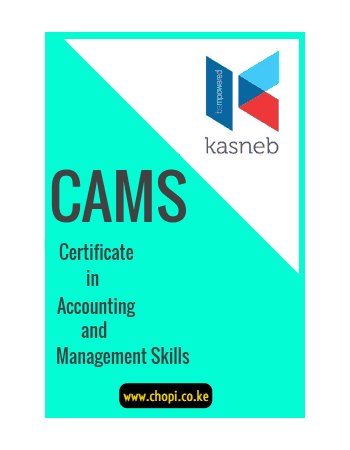# KASNEB CAMS – Fundamentals of Business Mathematics NotesCAMS LEVEL 1 NOTES

GENERAL OBJECTIVE
This paper is intended to equip the candidate with the requisite knowledge, skills and attitudes to apply basic mathematical concepts to solve problems in business and other environments.

LEARNING OUTCOMES
A candidate who passes this paper should be able to:

• Apply ratios, rates and proportions to interpret various scenarios
• Apply indices and logarithms in computations
• Use linear and matrix algebra to solve problems
• Apply basic calculus to solve problems
• Calculate measures of central tendency in statistics
• Construct and interpret basic graphs

• Ratios
• Rates
• Proportions
• Fractions
• Decimals
• Percentages

### Indices and Logarithms

• Notations
• Laws of indices
• Simplifying and solving indices
• Logarithms
• Change of base

### Linear Equations

• Linear equations in one unknown
• Linear equations in two unknowns
• Graphical solutions in two unknowns

### Matrix Algebra

• Notations
• Operations
• Solving a 2*2 matrix

### Sequences and Series

• Arithmetic progression(A.P):nth term, sum of first n terms
• Geometric progression (G.P): nth term, sum of first n terms

### 4.6 Commercial Mathematics

• Percentage profit and loss
• Simple interest and compound interest
• Appreciation and depreciation
• Basic income tax calculations
• Foreign exchange rates

### Calculus

• Basic differentiation
• Stationary points/turning points
• Basic integration

### Probability

• Definitions: event, experiment and outcome
• Types of events
• Rules/laws of probability
• Conditional probability

### Descriptive Statistics

• Data collection
• Data presentation; Frequency distribution table
• Mean, mode and median
• Variance and standard deviation

### Graphs

• Cartesian plane
• Linear graphs
• Graphs of simultaneous equations